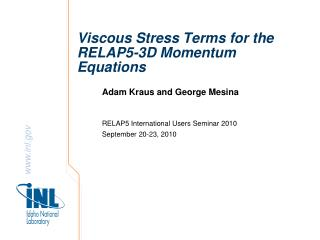Download PresentationViscous Stress Terms for the RELAP5-3D Momentum Equations

# Viscous Stress Terms for the RELAP5-3D Momentum Equations

Download Presentation## Viscous Stress Terms for the RELAP5-3D Momentum Equations

- - - - - - - - - - - - - - - - - - - - - - - - - - - E N D - - - - - - - - - - - - - - - - - - - - - - - - - - -
##### Presentation Transcript

1. Viscous Stress Terms for the RELAP5-3D Momentum Equations Adam Kraus and George Mesina RELAP5 International Users Seminar 2010 September 20-23, 2010

2. Outline of Presentation • Motivation for calculating viscous stresses • Previous work on liquid phase (Raymond Wang, Berkeley) • Compressibility and other changes for gas • Discretizations, boundary conditions • Discussion of results for laminar flow • Preliminary turbulent flow results • Future work

3. Motivation for Viscous Stress Implementation • Improved physical modeling • Greater accuracy for laminar flow regimes • Second-order effect • Accuracy • Improves convergence time • Better coupling of RELAP5 to CFD codes • Provide more accurate inlet flow field to CFD code

4. Previous Work (viscous) • Liquid phase viscous terms implemented • Cylindrical and Cartesian coordinates • Applies only to: • Laminar flow regimes • Incompressible fluids • Semi-implicit time advancement • Performed by Raymond Wang (Berkeley) • Subroutine “viscous” in flux3d

5. Gas Phase Viscous Calculations (viscousG) • Compressibility • Additional terms in Navier-Stokes Equations (NSE) • Usually ignored if Mach < 0.3 • No “bulk viscosity” term • Radial Velocity NSE • Incompressible • Compressible

6. Momentum Control Volume Grid (R-θ)

7. Momentum Control Volume Grid (R-Z)

8. Compressible Term Discretizations • Discretized with central differences • Example 1-direction discretization (pure partial derivative): • Example 2-direction discretization (mixed partial):

9. Subroutine for Gas Equation Viscous Terms • “No-slip” boundary condition (for viscous terms only) • Special handling developed for singularity at r=0 in cylindrical • Implemented in a new subroutine called “viscousG” • Corrects a couple programming errors in found viscous • Implements incompressible viscous term discretization with new naming convention • Implements compressibility term discretization • Implements boundary condiitons and r=0 handling

10. Test Model 1: Poiseuille Flow in Cylindrical Pipe • Inlet velocity profile: either plug or Poiseuille • Uniform mesh for each coordinate direction • 25 axial zones (L=2500m) • 9 radial zones (R=1m) • 1azimuthal zone

11. , H2O, 800°C

12. Test Model 2: Rectangular Duct • Inlet velocity profile: either plug or parabolic • Uniform mesh for each coordinate direction • 5 x-zones, 5 y-zones (width for both is 5m) • 5 axial zones (L=500m)

13. Rectangular Flow Results • Much more qualitative

14. Other Factors Affecting Velocity Profile • Significant Effects • Wall friction • Misapplied boundary condition (potentially) • Minor Effects • Change of fluid • Compressibility • “Numerical viscosity”

15. Turbulent Stress Modeling • RANS (general form) • represents previously calculated viscous terms (in tensor form) • is known as the Reynolds-stress tensor (must be modeled) • Selected turbulence model: Prandtl mixing length model where • U is the mean axial velocity, y is the distance from the boundary wall • Discretization:

16. Turbulent Stress Modeling • Modeling does not greatly reduce error of results • Better profile shape, follows power law • Problems must be run at very small time steps • Other differencing techniques should be investigated for better stability

17. Further Work • Apply only on wall cells in viscousG • Extend current work to nearly-implicit time advancement • Increase the maximum number of radial mesh increments (currently 9) • Stability analysis of numerical methods for turbulence calculations • Investigate more complex turbulence models

18. Acknowledgements • My mentor, Dr. George Mesina • The entire RELAP5 team • Idaho National Laboratory and the DOE Office of Science